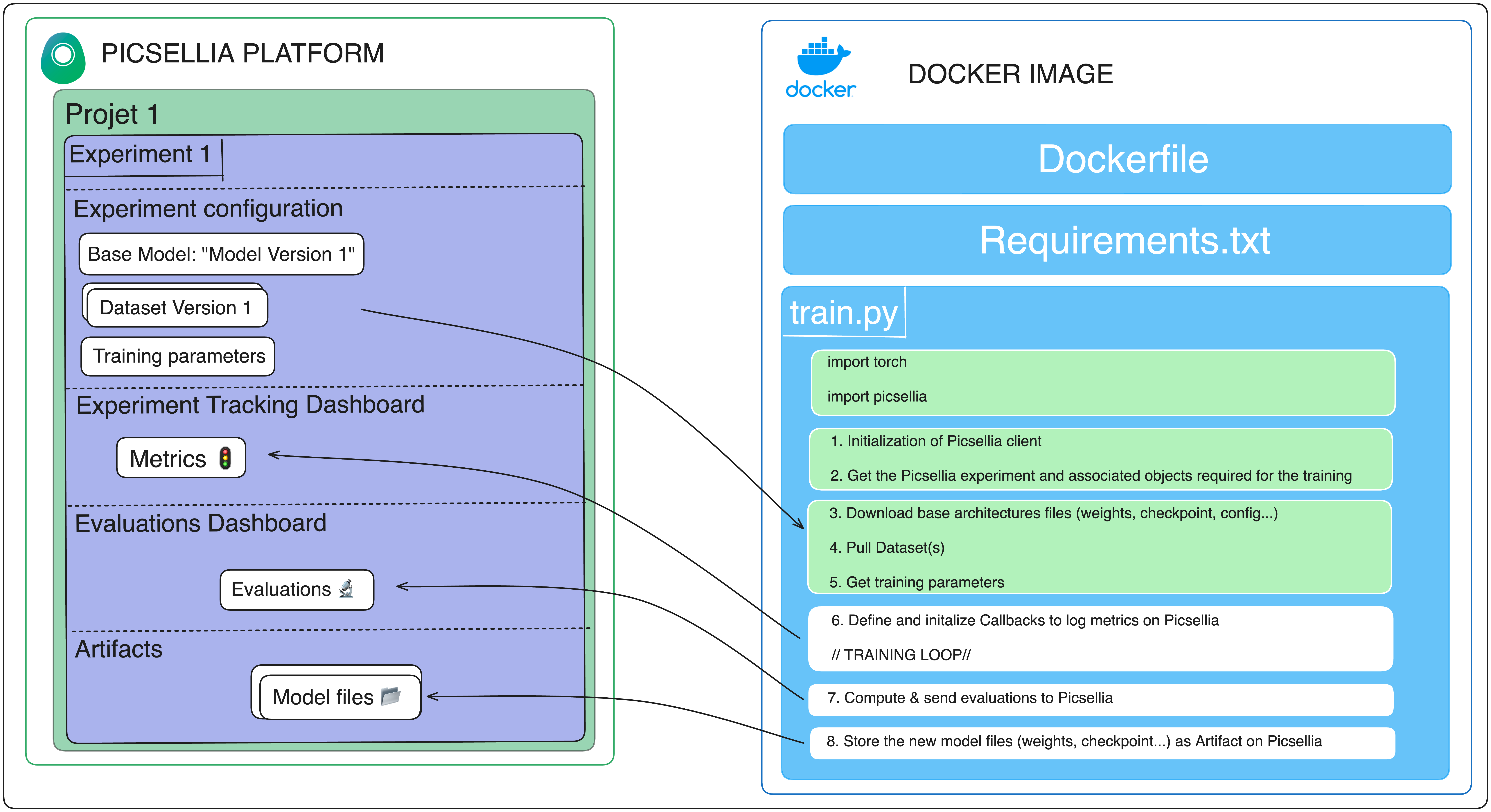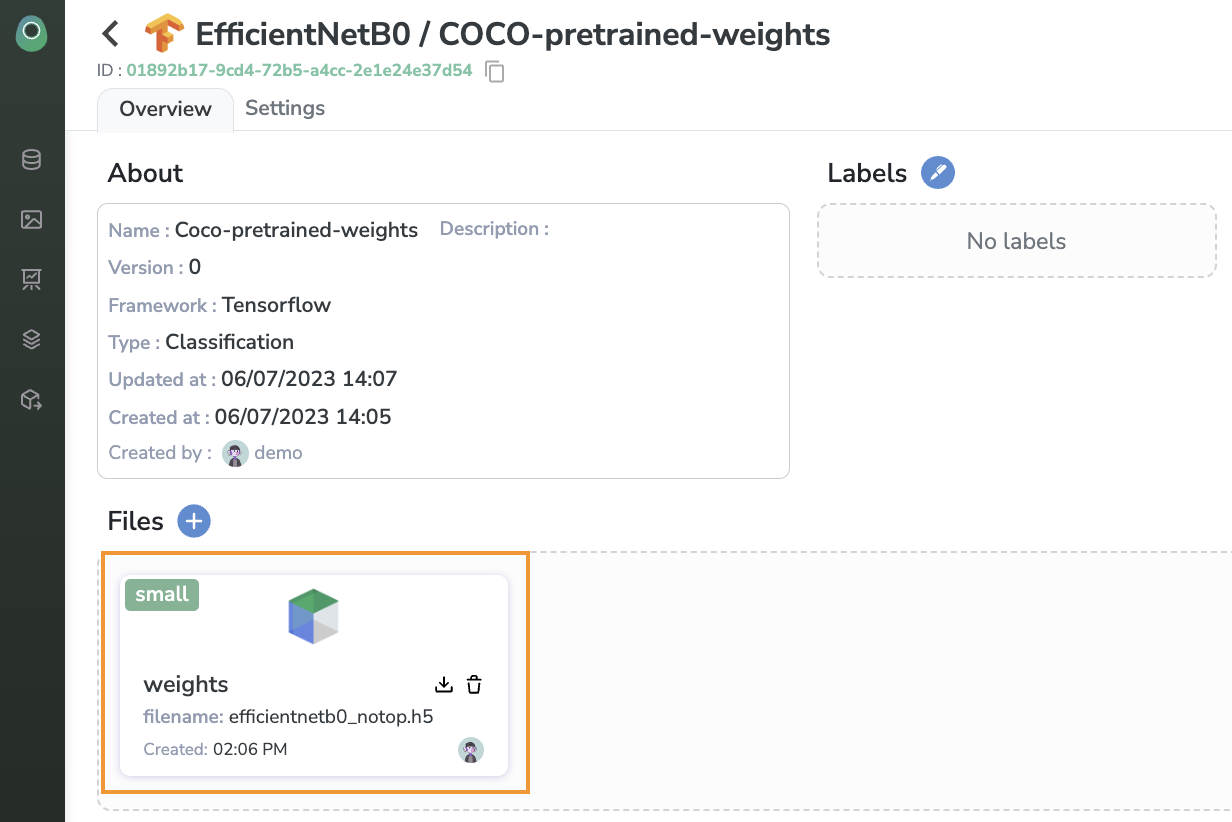# 3. Retrieve Data, files and parameters from Picsellia

It is now time to dissect your code and understand which object needs to be pulled from the Picsellia platform.

As a reminder, let's take another look at our schema:• The relevant `DatasetVerison`, which consist of `Asset` (check Datasetversion).
• The relevant weights files, including checkpoints, configuration files, and more (check Modelfile).
• The training parameters, such as the number of epochs and image size (check parameters).

# 1. Downloading the `DatasetVersion`

As we are doing a `CLASSIFICATION` use-case, we are going to download our `DatasetVersion` train and test in a folder structure way like this:

``````.
├── train/
│   ├── label_name_1/
│   │   ├── img1.jpg
│   │   ├── img2.jpg
│   │   └── ...
│   ├── label_name_2/
│   │   ├── img4.jpg
│   │   └── ...
│   └── ...
└── test/
├── label_name_1/
│   └── ...jpg
└── label_name_2/
└── ...jpg
``````

You can use the SDK to retrieve the `DatasetVersion` like this:

``````import os

client = Client(
api_token="XXXX",
organization_name="XXXX",
host="https://app.picsellia.com"
)

project = client.get_project("documentation-project")
experiment = project.get_experiment("exp-0-documentation")
datasets = experiment.list_attached_dataset_versions()

for dataset in datasets:
for label in dataset.list_labels():
os.makedirs(os.path.join(dataset.version, label.name))
assets = dataset.list_assets(
q=f"annotations.classifications.label.name = \"{str(label.name)}\""
``````

If you want to understand more about Picsellia Asset and Export Annotations Fileoptions you can check our Client reference (for example, you can download your dataset in COCO or YOLO format).

For our custom EfficientNetB0 model, we stored our weights under the name weights.``````# ...

base_model = experiment.get_base_model_version()
base_model_weights = base_model.get_file('weights')
``````

# 3. Retrieving the training parameters

Our training script is taking some parameters as inputs, allowing the user to customize the training. So we need to retrieve the parameters defined in the Picsellia `Experiment` and pass them to the training script.

``````# ...

parameters = experiment.get_log(name='parameters').data
IMG_SIZE = parameters['image_size']
batch_size = parameters['batch_size']
``````

Parameters are sent by Picselia as a dictionary, for instance:

``````parameters = {
'epochs': 50,
'batch_size': 64,
'image_size': 224
}
``````

So now you just need to assign each parameter to its related variable in your training script!

# 4. Wrapping up

Now your training script should look something like this.

``````from tensorflow.keras.applications import EfficientNetB0
from tensorflow.keras.models import Sequential
from tensorflow.keras import layers
import tensorflow as tf
import tensorflow_datasets as tfds
from picsellia import Client
import os

## Initialization of the Picsellia client
client = Client(
api_token="XXXXXXX",
organization_name="my_organization",
host="https://app.picsellia.com"
)

## Retrieve the Picsellia experiment that needs to be trained
project = client.get_project("documentation_project")
experiment = project.get_experiment("exp-0-documentation")

## Download the datasets from Picsellia and organize the data on the infrastrcture drive
datasets = experiment.list_attached_dataset_versions()
for dataset in datasets:
for label in dataset.list_labels():
os.makedirs(os.path.join(dataset.version, label.name))

base_model = experiment.get_base_model_version()
base_model_weights = base_model.get_file('weights')

## Get experiment parameters that will define the training
parameters = experiment.get_log(name='parameters').data
IMG_SIZE = parameters['image_size']
batch_size = parameters['batch_size']

## Hardware connection
try:
tpu = tf.distribute.cluster_resolver.TPUClusterResolver.connect()
print("Device:", tpu.master())
strategy = tf.distribute.TPUStrategy(tpu)
except ValueError:
print("Not connected to a TPU runtime. Using CPU/GPU strategy")
strategy = tf.distribute.MirroredStrategy()

## Keras preprocessing
size = (IMG_SIZE, IMG_SIZE)

train_img_gen = tf.keras.preprocessing.image.ImageDataGenerator(rescale=1./255, rotation_range=20)
test_img_gen = tf.keras.preprocessing.image.ImageDataGenerator(rescale=1./255)
ds_train = next(train_img_gen.flow_from_directory('train', target_size=size))
ds_test = next(test_img_gen.flow_from_directory('test', target_size=size))

NUM_CLASSES = len(experiment.get_dataset('train').list_labels())
``````